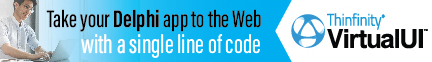DelphiBasicsDoubleType A floating point type supporting about 15 digits of precision System unit
 type Double;
Description
The Double type is the general purpose floating point type in Delphi. It strikes the balance between capacity/precision versus storage/performance.

It supports approximately 15 digits of precision in a range from 2.23 x 10-308 to 1.79 x 10308.
Notes
The Single type is smaller and faster, but with reduced capacity and precision.

The Extended type has the highest capacity and precision, but biggest storage and worst performance.

A Double set to its highest value is treated as Infinity.
Related commands
 Currency A decimal type with 4 decimal points used for financial values Extended The floating point type with the highest capacity and precision Single The smallest capacity and precision floating point type
Author linksDownload this web site as a Windows program.

 Example code : Showing the precision and capacity of Double values var   account1, account2, account3, account4 : Double; begin  account1 := 0.1234567890123456789;      // 20 decimal places  account2 := 1.234567890123456789E308;   // Highest exponent value   account3 := account1 + account2;  account4 := 9.9E308;                    // Treated as infinite   ShowMessage('Account1 = '+FloatToStr(account1));   ShowMessage('Account2 = '+FloatToStr(account2));   ShowMessage('Account3 = '+FloatToStr(account3));   ShowMessage('Account4 = '+FloatToStr(account4)); end; Show full unit code Account1 = 0.123456789012346   Account2 = 1.23456789012346E308   Account3 = 1.23456789012346E308   Account4 = INF
 Delphi Programming © Neil Moffatt 2002 - 2020. All rights reserved.  |  Home Page